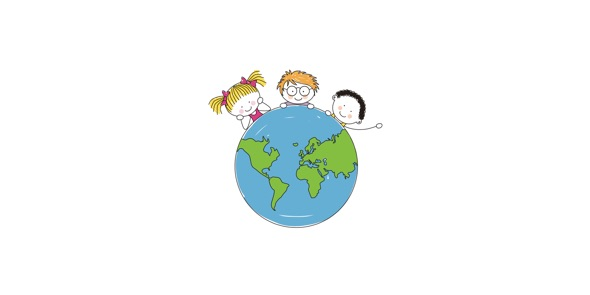# 7th Grade Q4 2012 Pre Test

15 Questions | Total Attempts: 100Settings• 1.
In Excel, the worksheet cell with the dark border that is ready for data entry is called ______________.
• A.

The formula bar

• B.

The active cell

• C.

The cursor

• D.

Cell reference

• 2.
When in an internet browser, like Internet Explorer, the area in which you type a web address is called ______________.
• A.

• B.

The formula bar

• C.

The location bar

• D.

The web bar

• 3.
Left, Center and Right are all examples of what?
• A.

Directions

• B.

Alignment

• C.

Location

• D.

Font

• 4.
The AutoFill feature is part of what program?
• A.

Microsoft Word

• B.

Dragon Naturally Speaking

• C.

Microsoft Excel

• D.

PhotoStory 3

• 5.
A financial plan that helps you plan for future expenses(\$) is called a what? Please use only one word.
• 6.
The place where a column and a row intersect is called a what?
• A.

Box

• B.

Intersection

• C.

Table

• D.

Cell

• 7.
Another word for a cell's name
• A.

Is the cell's cell reference

• B.

Is the cell's location

• C.

• D.

Would be like A2

• 8.
A column
• A.

Is in a newspaper

• B.

Goes up and down

• C.

Are in front of some houses

• D.

Go left and right

• 9.
• A.

The home screen

• B.

• C.

The desktop

• D.

The beginning screen

• 10.
A formula
• A.

Is an equation you use in Algebra class to calculate a math problem.

• B.

Is an equation the computer uses to calculate a math problem

• C.

Is a math problem that uses letters when you don't know the answer.

• D.

Is a math problem that you'll learn about in high school

• 11.
Folders
• A.

Are left in class so you don't have to bring your handouts back.

• B.

Are used to help keep your files organized

• C.

Can't be created at school because that function is blocked

• D.

Should only be used if you are unorganized

• 12.
Margins are
• A.

Equal to 2 inches

• B.

The white space at the edge of the paper

• C.

The number of mistakes your allowed to make

• D.

None of the above

• 13.
The server stores your files on the actual computer in front of you.
• A.

True

• B.

False

• 14.
It is ok to share personal information on the internet.
• A.

True

• B.

False

• 15.
It is ok to share private information on the internet.
• A.

True

• B.

False

Related Topics